# Solved Problems Signal Detection Theory 1 Compare the

• Slides: 9
Download presentationSolved Problems: Signal Detection Theory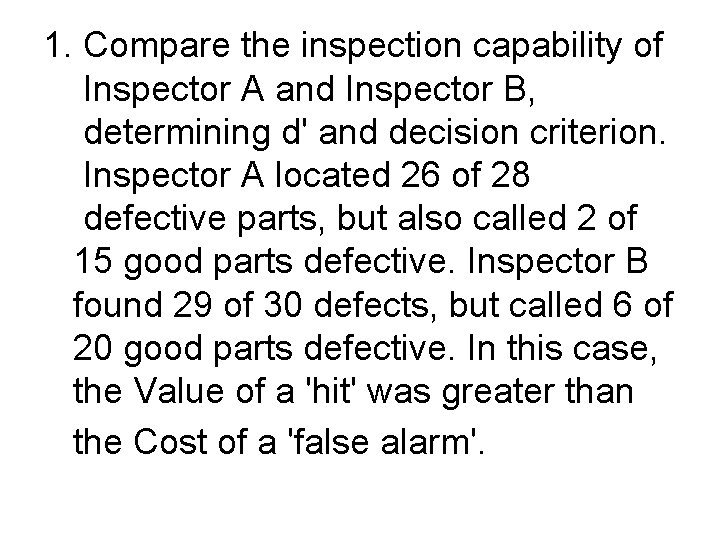1. Compare the inspection capability of Inspector A and Inspector B, determining d' and decision criterion. Inspector A located 26 of 28 defective parts, but also called 2 of 15 good parts defective. Inspector B found 29 of 30 defects, but called 6 of 20 good parts defective. In this case, the Value of a 'hit' was greater than the Cost of a 'false alarm'.Inspector A: HR = 26/28 =. 93; Z = 1. 48 FAR = 2/15 =. 13; Z = 1. 13 We draw a picture of the model, and determine that the decision criterion is located between the means of the two distributions*; therefore, we add the Z-scores from each distribution to compute d'. Note that this inspector is fairly neutral (neither liberal or conservative). d' = 1. 48 + 1. 13 = 2. 61 Inspector B: HR = 29/30 =. 97; Z = 1. 88 FAR = 6/20 =. 3; Z =. 52 Similarly, we add the two Z-scores to compute d'. Note that, if FAR had been greater than. 5, we would have to subtract one of the Z-values. This inspector was somewhat more liberal than inspector A. d' = 1. 88 +. 52 = 2. 40. Thus, Inspector A was a bit better discriminator. If the value of a hit is greater than the cost of a FA, then we seek a very liberal inspector. We might decide to go with inspector B, if the cost of a FA is small enough and the value of a Hit is great enough.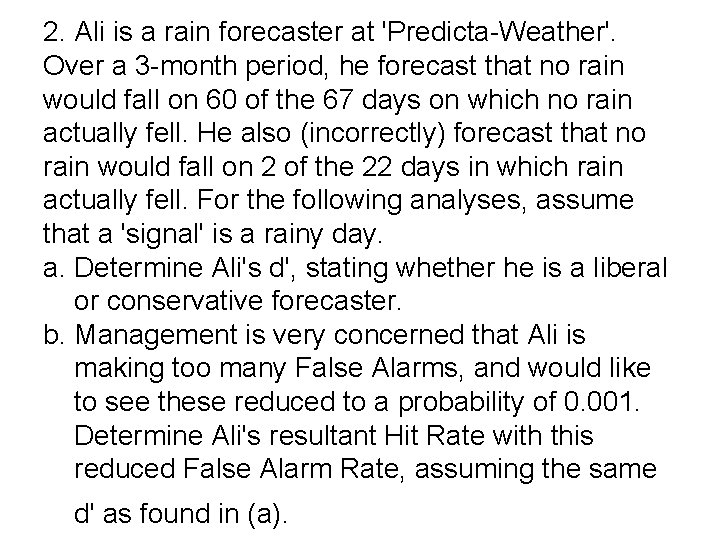2. Ali is a rain forecaster at 'Predicta-Weather'. Over a 3 -month period, he forecast that no rain would fall on 60 of the 67 days on which no rain actually fell. He also (incorrectly) forecast that no rain would fall on 2 of the 22 days in which rain actually fell. For the following analyses, assume that a 'signal' is a rainy day. a. Determine Ali's d', stating whether he is a liberal or conservative forecaster. b. Management is very concerned that Ali is making too many False Alarms, and would like to see these reduced to a probability of 0. 001. Determine Ali's resultant Hit Rate with this reduced False Alarm Rate, assuming the same d' as found in (a).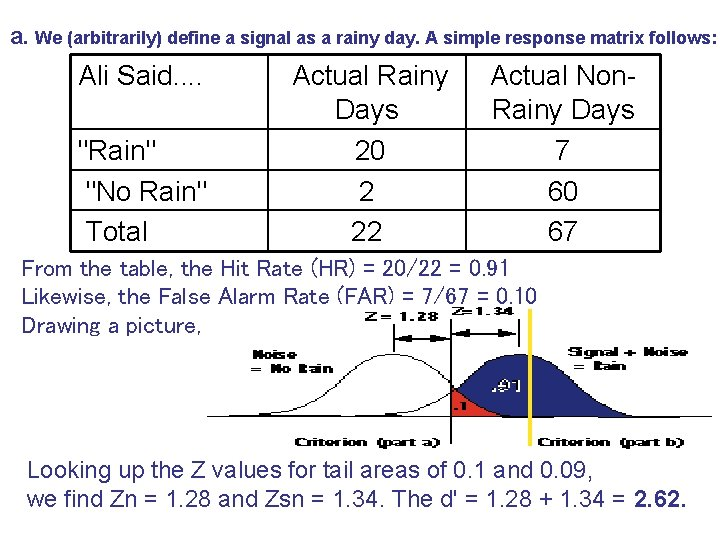a. We (arbitrarily) define a signal as a rainy day. A simple response matrix follows: Ali Said. . "Rain" "No Rain" Total Actual Rainy Days 20 2 22 Actual Non. Rainy Days 7 60 67 From the table, the Hit Rate (HR) = 20/22 = 0. 91 Likewise, the False Alarm Rate (FAR) = 7/67 = 0. 10 Drawing a picture, we see the following: Looking up the Z values for tail areas of 0. 1 and 0. 09, we find Zn = 1. 28 and Zsn = 1. 34. The d' = 1. 28 + 1. 34 = 2. 62.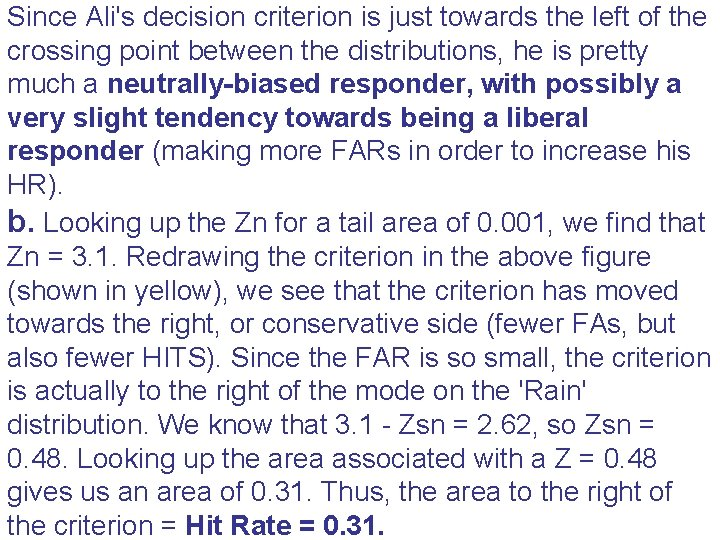Since Ali's decision criterion is just towards the left of the crossing point between the distributions, he is pretty much a neutrally-biased responder, with possibly a very slight tendency towards being a liberal responder (making more FARs in order to increase his HR). b. Looking up the Zn for a tail area of 0. 001, we find that Zn = 3. 1. Redrawing the criterion in the above figure (shown in yellow), we see that the criterion has moved towards the right, or conservative side (fewer FAs, but also fewer HITS). Since the FAR is so small, the criterion is actually to the right of the mode on the 'Rain' distribution. We know that 3. 1 - Zsn = 2. 62, so Zsn = 0. 48. Looking up the area associated with a Z = 0. 48 gives us an area of 0. 31. Thus, the area to the right of the criterion = Hit Rate = 0. 31.Try to solve this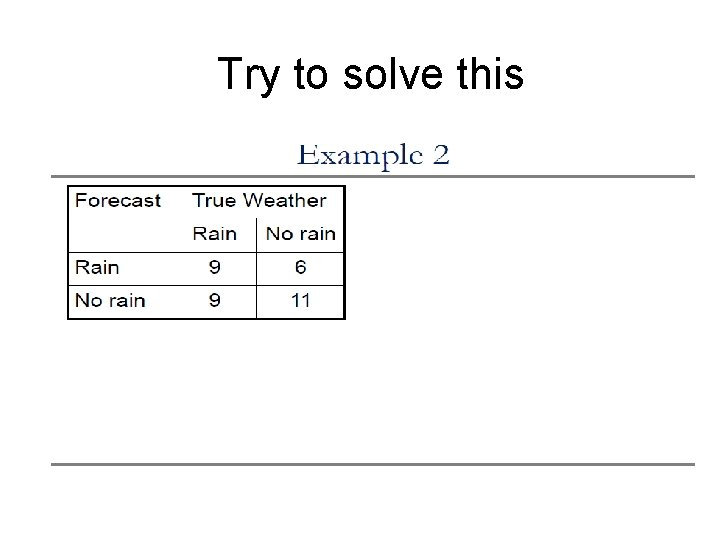Try to solve this